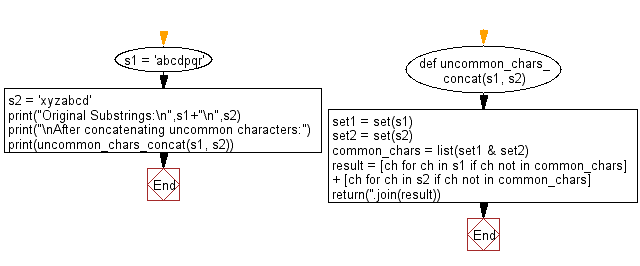﻿ Python: Create a string from two given strings concatenating uncommon characters of the said strings - w3resource# Python: Create a string from two given strings concatenating uncommon characters of the said strings

## Python String: Exercise-70 with Solution

Write a Python program to create a string from two given strings concatenating uncommon characters of the said strings.

Sample Solution:-

Python Code:

``````def uncommon_chars_concat(s1, s2):

set1 = set(s1)
set2 = set(s2)

common_chars = list(set1 & set2)
result = [ch for ch in s1 if ch not in common_chars] + [ch for ch in s2 if ch not in common_chars]
return(''.join(result))

s1 = 'abcdpqr'
s2 = 'xyzabcd'
print("Original Substrings:\n",s1+"\n",s2)
print("\nAfter concatenating uncommon characters:")
print(uncommon_chars_concat(s1, s2))
```
```

Sample Output:

```Original Substrings:
abcdpqr
xyzabcd

After concatenating uncommon characters:
pqrxyz
```

Pictorial Presentation:Flowchart:## Visualize Python code execution:

The following tool visualize what the computer is doing step-by-step as it executes the said program:

Python Code Editor:

Have another way to solve this solution? Contribute your code (and comments) through Disqus.

What is the difficulty level of this exercise?

Test your Programming skills with w3resource's quiz.

﻿

## Python: Tips of the Day

Check if a given key already exists in a dictionary:

In is the intended way to test for the existence of a key in a dict.

```d = {"key1": 10, "key2": 23}

if "key1" in d:
print("this will execute")

if "nonexistent key" in d:
print("this will not")
```

If you wanted a default, you can always use dict.get():

```d = dict()

for i in range(100):
key = i % 10
d[key] = d.get(key, 0) + 1
```

and if you wanted to always ensure a default value for any key you can either use dict.setdefault() repeatedly or defaultdict from the collections module, like so:

```from collections import defaultdict

d = defaultdict(int)

for i in range(100):
d[i % 10] += 1
```

but in general, the in keyword is the best way to do it.

Ref: https://bit.ly/2XPMRyz